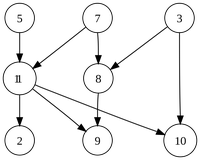# Glossary

## Directed graph

A directed graph is a graph whose edges all have an orientation and are thus represented by arrows instead of segments.

Directed graphs are useful when the relationship connecting nodes works in one direction but not necessarily the opposite direction. For example, in an overlap graph, strings $s$ and $t$ are connected with a directed edge if a suffix of $s$ is equal to a prefix of $t$. The opposite case is not necessarily true, which is why directed edges are appropriate for the overlap graph.

The figure below illustrates a simple directed graph whose nodes are labeled with integers. This directed graph is a particular type of graph containing no cycles, fittingly called a directed acyclic graph.